# Olympiad Test: Percentage - 2

## 20 Questions MCQ Test Mathematics Olympiad Class 7 | Olympiad Test: Percentage - 2

Description
Attempt Olympiad Test: Percentage - 2 | 20 questions in 40 minutes | Mock test for Class 7 preparation | Free important questions MCQ to study Mathematics Olympiad Class 7 for Class 7 Exam | Download free PDF with solutions
QUESTION: 1

### Goods were bought for Rs 600 and sold the same for Rs 688.50 at a credit of 9 months and thus gaining 2%. The rate of interest per annum is:

Solution:

S.P. = 102% of Rs 600 = Rs 612
Now, P.W. = Rs 612 and sum = Rs 688.50
∴ T.D. = Rs (688.50 – 612) = Rs 76.50
Thus, S.I. on Rs 612 for 9 months is Rs 76.50
∴ Rate = [(100 × 76.50)/ (612 × 3/4)] %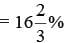QUESTION: 2

### The true discount on a bill due 9 months hence at 16% per annum is Rs 189. The amount of the bill is:

Solution:

Let P.W. be Rs x.
Then, S.I. on Rs x at 16% for 9 months = Rs 189
∴  x × 16 × 9/12×1/100
= 189 or x = 1575
∴ P.W. = Rs 1575
∴ Sum due
= P.W. + T.D. = Rs (1575 + 189)
= Rs 1764

QUESTION: 3

### A man buys a watch for Rs 1950 in cash and sells it for Rs 2200 at a credit of 1 year. If the rate of interest is 10% per annum, the man:

Solution:

S.P. = P.W. of Rs 2200 due 1 year hence
= Rs [(2200 × 100)/ 100 + (10 × 1)]
= Rs 2000
∴ Gain = Rs (2000 – 1950) = Rs 50

QUESTION: 4

The true discount on Rs 1760 due after a certain time at 12% per annum is Rs 160. The time after which it is due is:

Solution:

P.W. = Rs (1760 – 160) = Rs 1600
∴ S.I. on Rs 1600 at 12% is Rs 160
∴ Time = (100 × 160)/ (1600 × 12) = 5/6
years = (5/6 × 12 months) = 10 months

QUESTION: 5

The present worth of Rs 2310 due 2.5 years hence, the rate of interest being 15% per annum, is:

Solution:

P.W. = Rs [(100 × 2310)/ (100 + 15 × 5/12)
= Rs 1680

QUESTION: 6

Rs 20 is the true discount on Rs 260 due after a certain time. What will be the true discount on the same sum due after half of the former time, the rate of interest being the same?

Solution:

S.I. on Rs (260 – 20) for a given time = Rs 20
S.I. on Rs 240 for half the time = Rs 10.
T.D. on Rs 250 = Rs 10.
∴ T.D. on Rs 260 = Rs [(10/250) × 260]
= Rs 10.40

QUESTION: 7

The interest on Rs 750 for 2 years is the same as the true discount on Rs 960 due 2 years hence. If the rate of interest is the same in both cases, it is:

Solution:

S.I. on Rs 750 = T.D. on Rs 960.
This means P.W. of Rs 960 due 2 years
hence is Rs 750.
∴ T.D. = Rs (960 – 750) = Rs 210.
Thus, S.I. on Rs 750 for 2 years is Rs 210.
∴ Rate = [(100 × 210)/ (750 × 2)] %
= 14%

QUESTION: 8

The simple interest and the true discount on a certain sum for a given time and at a given rate are Rs 85 and Rs 80 respectively. The sum is:

Solution:

Sum = (S.I. × T.D.)/ (S.I.) – (T.D.)
= 85 × 80/ (85 – 80) = Rs 1360

QUESTION: 9

The present worth of Rs 1404 due in two equal half-yearly instalments at 8% per annum simple interest is:

Solution:

Required sum = P.W. of Rs 702 due 6 months + P.W. of Rs 702 due 1 year hence
= [(100 × 702)/(100 + 8 × 1/2)
+ (100 × 702)/(100 + 8 × 1)]
= Rs (675 + 650) = Rs 1325

QUESTION: 10

If the true discount on a sum due 2 years hence at 14% per annum be Rs 168, the sum due is:

Solution:

P.W. = (100 × T.D.)/ R × T
= (100 × 168)/14 × 2 = 600
∴ Sum = (P.W. + T.D.) = Rs (600 + 168)
= Rs 768

QUESTION: 11

The population of a town has increased from 60,000 to 65,000. Find the increase percent.

Solution:

Increase in population = 65,000 – 60,000
= 5,000
Percentage Increase
= (5,000/60,000) × 100%
= (25/3) % =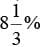QUESTION: 12

Ram’s salary is increased from Rs 630 to Rs 700. Find the increase percent.

Solution:

Increase in salary = Rs 700 – Rs 630 = Rs 70
Percent Increase = (70/630) × 100%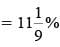QUESTION: 13

In an election of two candidates, the candidate who gets 41% is rejected by a majority of 2412 votes. Find the total number of votes polled.

Solution:

(59% – 41%) = 18% ≡ 2412
∴ 100% ≡ (2412/18) × 100 = 13400

QUESTION: 14

A man loses 12.5% of his money and after spending 70% of the remainder he left with Rs 210. How much did he have at first?

Solution:

His Initial money == Rs 800

QUESTION: 15

3.5% of income is taken as tax and 12.5% of the remaining is saved. This leaves Rs 4,053 to spend. What is the income?

Solution: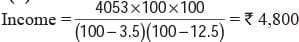QUESTION: 16

If 2 litres of water is evaporated on boiling from 8 litres of sugar solution containing 5% sugar, find the percentage of sugar in the remaining solution.

Solution:

Sugar in the original solution
= 5% of 8 litres = 0.4 litres
After evaporation of 2 litres of water, the quantity of remaining solution
= 8 – 2 = 6 litres
∴ Required percentage of sugar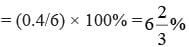QUESTION: 17

Due to fall in manpower, the production in a factory decreases by 25%. By what percent should be working hour be increased to restore the original production?

Solution:

Required percentage increase in working hours
= [25/(100 – 25)] × 100 = 100/3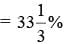QUESTION: 18

12% of a certain sum of money is Rs 43.5 Find the sum.

Solution: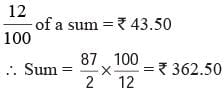QUESTION: 19

Two numbers are respectively 20% and 50% more than the third. What percent is the first of the second?

Solution:

Required value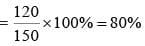QUESTION: 20

Two numbers are respectively 20% and 25% of a third number. What percent is the first of the second?

Solution:

Required value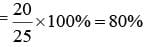Use Code STAYHOME200 and get INR 200 additional OFF Use Coupon Code